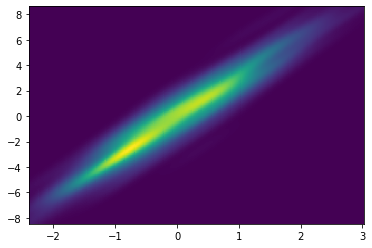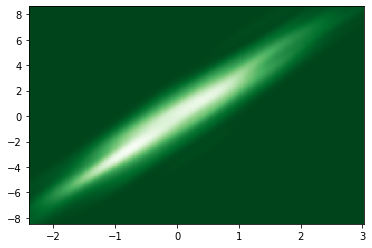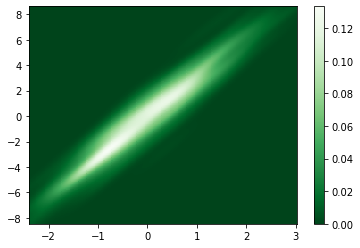# Density Plot with Matplotlib

This post aims to display density plots built with matplotlib and shows how to calculate a 2D kernel density estimate.

Let’s consider that you want to study the relationship between 2 numerical variables with a lot of points. Then you can consider the number of points on each part of the plotting area and thus calculate a 2D kernel density estimate. It is like a smoothed histogram. Instead of a point falling into a particular bin, it adds a weight to surrounding bins. This plot is inspired from this stack overflow question. See this page to custom the color palette.

``````# libraries
import matplotlib.pyplot as plt
import numpy as np
from scipy.stats import kde

# create data
x = np.random.normal(size=500)
y = x * 3 + np.random.normal(size=500)

# Evaluate a gaussian kde on a regular grid of nbins x nbins over data extents
nbins=300
k = kde.gaussian_kde([x,y])
xi, yi = np.mgrid[x.min():x.max():nbins*1j, y.min():y.max():nbins*1j]
zi = k(np.vstack([xi.flatten(), yi.flatten()]))

# Make the plot
plt.show()

# Change color palette
plt.show()``````You can add a color bar easily using `colorbar()` function.

``````# Add color bar👋 This document is a work by Yan Holtz. Any feedback is highly encouraged. You can fill an issue on Github, drop me a message onTwitter, or send an email pasting `yan.holtz.data` with `gmail.com`.